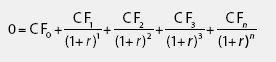# internal rate of return

(redirected from Dollar Weighted Rate of Return)
Also found in: Dictionary.

## Internal rate of return (IRR)

Dollar-weighted rate of return. Discount rate at which net present value (NPV) of an investment is zero. The rate at which a bond's future cash flows, discounted back to today, equal its price.

## Internal Rate of Return

The discount rate at which the cash inflow on an investment equals its cash outflow. That is, the internal rate of return is the return necessary for the present value of an investment to equal what one spends in making the investment. Importantly, the internal rate of return accounts for inflation. See also: Yield to maturity.

## internal rate of return (IRR)

The rate of discount on an investment that equates the present value of the investment's cash outflows with the present value of the investment's cash inflows. Internal rate of return is analogous to yield to maturity for a bond.

## internal rate of return

see DISCOUNTED CASH FLOW.

## internal rate of return

see DISCOUNTED CASH FLOW.

## internal rate of returnThe annual rate of earnings on an investment equates the value of cash returns with the cash invested,taking into consideration the power of compounding interest.The following formula requires a trial-and-error method for solution. The fallacy with the method is its assumption that all cash flows are reinvested at the internal rate of return.

Using this formula,CF is the cash flow in each period,with one subpart of the formula for each investment year until you reach n,the final year of the investment.You have to solve for R, the annual rate, by trial and error.

Site: Follow: Share:
Open / Close